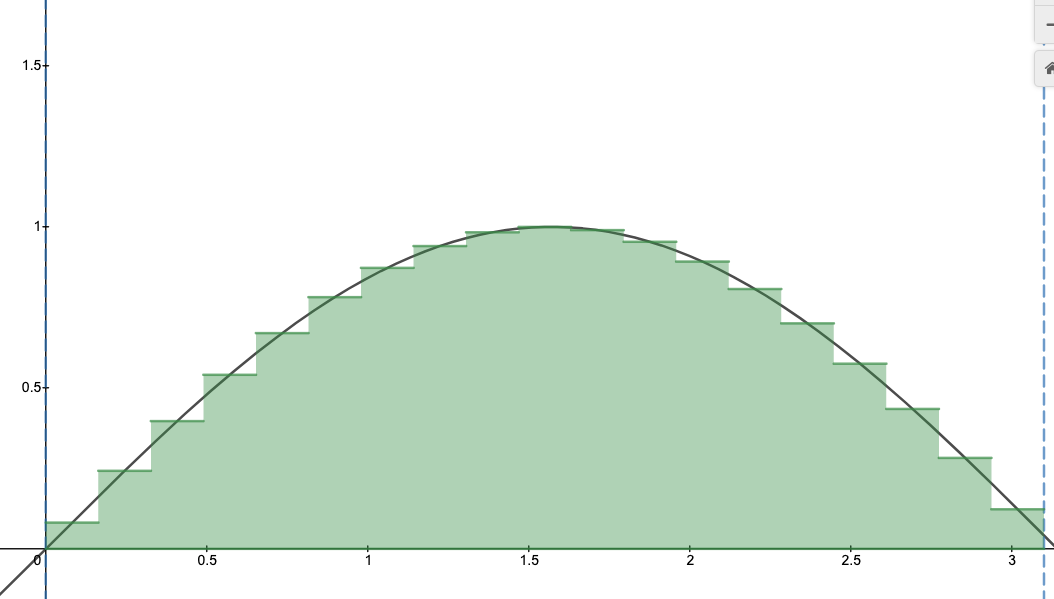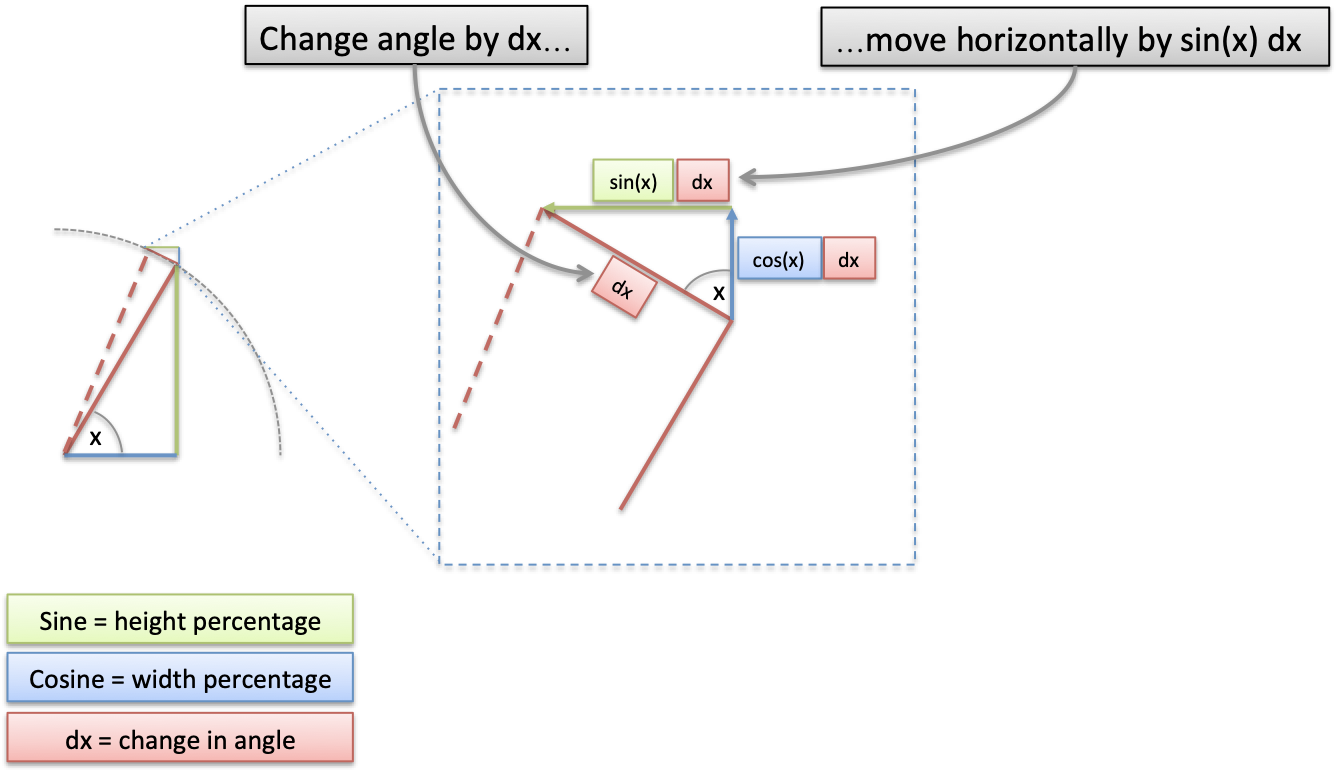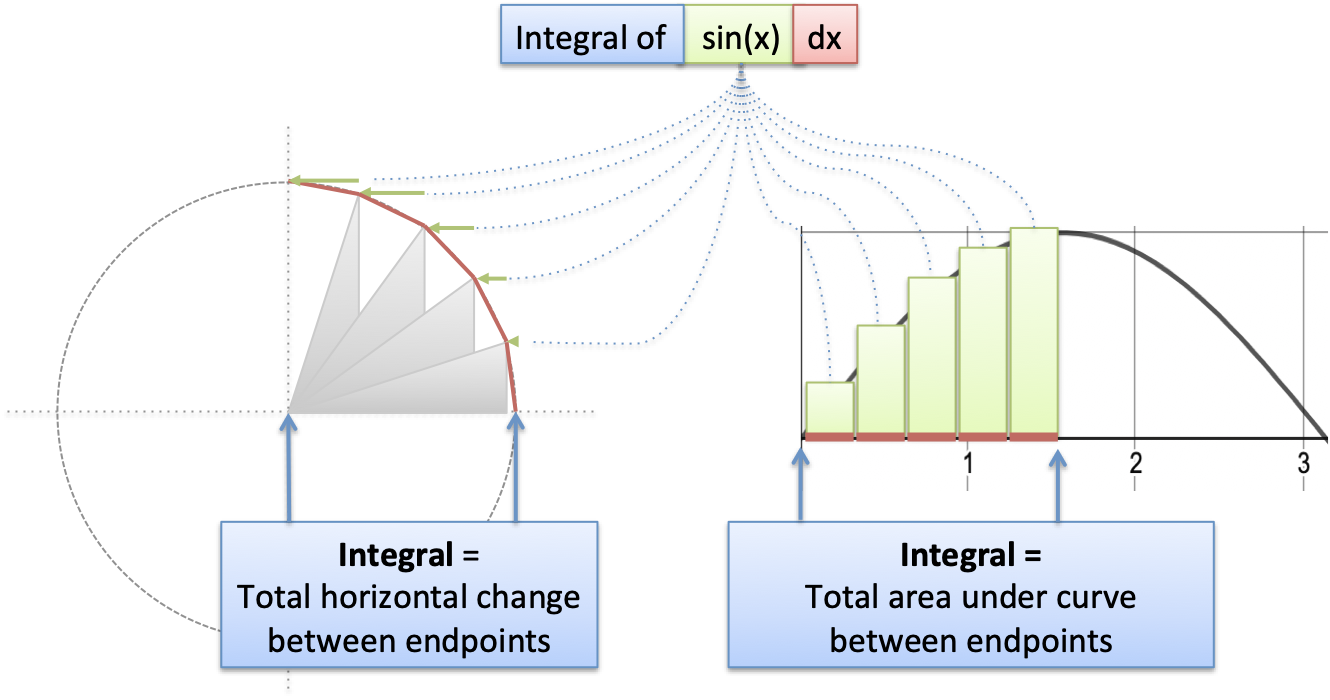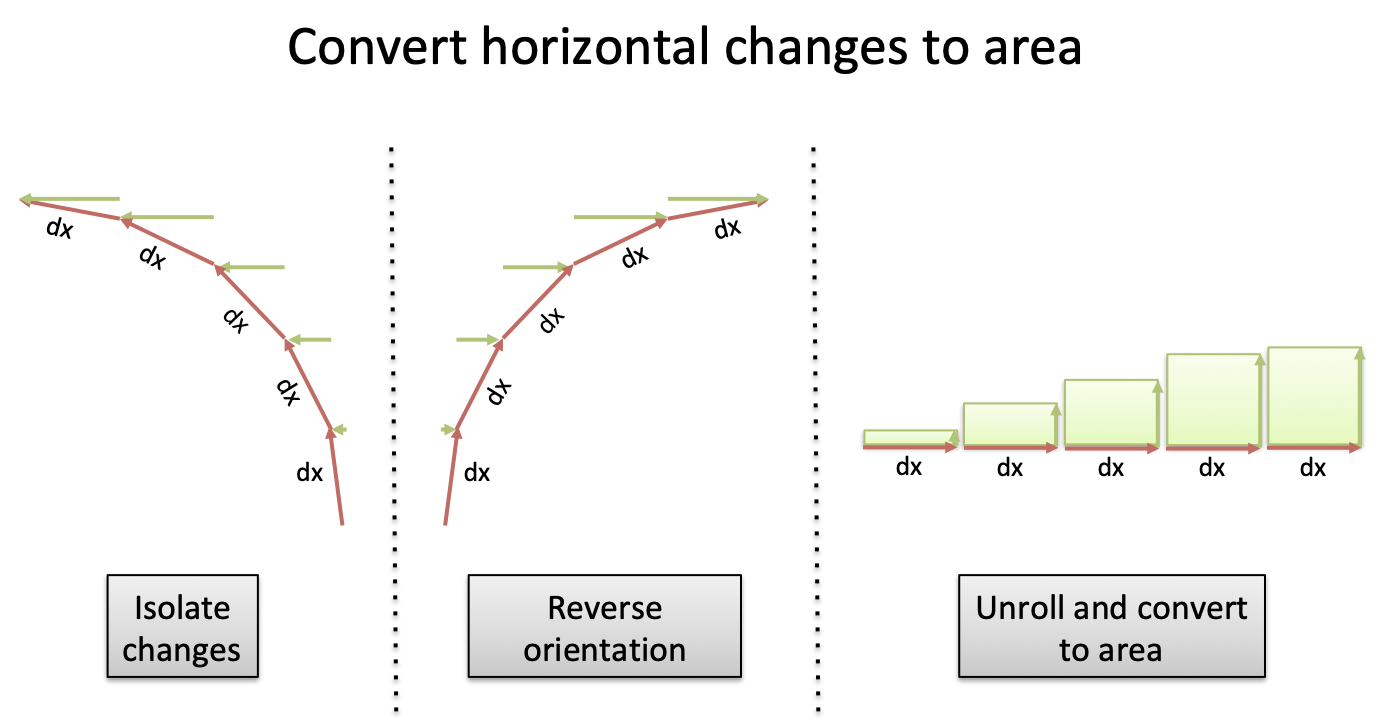# Integral of Sin(x): Geometric Intuition

You're minding your own business when some punk asks what the integral of $\sin(x)$ means. Your options:

• Pretend to be asleep (except not in the library again)
• Canned response: "As with any function, the integral of sine is the area under its curve."
• Geometric intuition: "The integral of sine is the horizontal distance along a circular path."

Option 1 is tempting, but let's take a look at the others.

## Why "Area Under the Curve" is Unsatisfying

Describing an integral as "area under the curve" is like describing a book as a list of words. Technically correct, but misses the message and I suspect you haven't done the assigned reading.Unless you're trapped in LegoLand, integrals mean something besides rectangles.

## Decoding the Integral

My calculus conundrum was not having an intuition for all the mechanics.

When we see:

$\int \sin(x) dx$

We can call on a few insights:

• The integral is just fancy multiplication. Multiplication accumulates numbers that don't change (3 + 3 + 3 + 3). Integrals add up numbers that might change, based on a pattern (1 + 2 + 3 + 4). But if we squint our eyes and pretend items are identical we have a multiplication.

• $\sin(x)$ just a percentage. Yes, it's also fancy curve with nice properties. But at any point (like 45 degrees), it's a single percentage from -100% to +100%. Just regular numbers.

• $dx$ is a tiny, infinitesimal part of the path we're taking. 0 to $x$ is the full path, so $dx$ is (intuitively) a nanometer.

Ok. With those 3 intuitions, our rough (rough!) conversion to Plain English is:

The integral of sin(x) multiplies our intended path length (from 0 to x) by a percentage

We intend to travel a simple path from 0 to x, but we end up with a smaller percentage instead. (Why? Because $\sin(x)$ is usually less than 100%). So we'd expect something like 0.75x.

In fact, if $\sin(x)$ did have a fixed value of 0.75, our integral would be:

$\int \text{fixedsin}(x) = \int 0.75 \ dx = 0.75 \int dx = 0.75x$

But the real $\sin(x)$, that rascal, changes as we go. Let's see what fraction of our path we really get.

## Visualize The Change in Sin(x)

Now let's visualize $\sin(x)$ and its changes:Here's the decoder key:

• $x$ is our current angle in radians. On the unit circle (radius=1), the angle is the distance along the circumference.

• $dx$ is a tiny change in our angle, which becomes the same change along the circumference (moving 0.01 units in our angle moves 0.01 along the circumference).

• At our tiny scale, a circle is a a polygon with many sides, so we're moving along a line segment of length $dx$. This puts us at a new position.

With me? With trigonometry, we can find the exact change in height/width as we slide along the circle by $dx$.

By similar triangles, our change just just our original triangle, rotated and scaled.

• Original triangle (hypotenuse = 1): height = $\sin(x)$, width = $\cos(x)$
• Change triangle (hypotenuse = dx): height = $\sin(x) dx$, width = $\cos(x) dx$

Now, remember that sine and cosine are functions that return percentages. (A number like 0.75 doesn't have its orientation. It shows up and makes things 75% of their size in whatever direction they're facing.)

So, given how we've drawn our Triangle of Change, $\sin(x) dx$ is our horizontal change. Our plain-English intuition is:

The integral of sin(x) adds up the horizontal change along our path

## Visualize The Integral Intuition

Ok. Let's graph this bad boy to see what's happening. With our "$\sin(x) dx$ = tiny horizontal change" insight we have:As we circle around, we have a bunch of $dx$ line segments (in red). When sine is small (around x=0) we barely get any horizontal motion. As sine gets larger (top of circle), we are moving up to 100% horizontally.

Ultimately, the various $\sin(x) dx$ segments move us horizontally from one side of the circle to the other.

A more technical description:

$\int_0^x \sin(x) dx = \text{horizontal distance traveled on arc from 0 to x}$

Aha! That's the meaning. Let's eyeball it. When moving from $x=0$ to $x=\pi$ we move exactly 2 units horizontally. It makes complete sense in the diagram.

## The Official Calculation

Using the Official Calculus Fact that $\int \sin(x) dx = -\cos(x)$ we would calculate:

$\int_0^\pi \sin(x) dx = -\cos(x) \Big|_0^\pi = -\cos(\pi) - -\cos(0) = -(-1) -(-1) = 1 + 1 = 2$

Yowza. See how awkward it is, those double negations? Why was the visual intuition so much simpler?

Our path along the circle ($x=0$ to $x=\pi$) moves from right-to-left. But the x-axis goes positive from left-to-right. When convert distance along our path into Standard Area™, we have to flip our axes:Our excitement to put things in the official format stamped out the intuition of what was happening.

## Fundamental Theorem of Calculus

We don't really talk about the Fundamental Theorem of Calculus anymore. (Is it something I did?)

Instead of adding up all the tiny segments, just do: end point - start point.

The intuition was staring us in the face: $\cos(x)$ is the anti-derivative, and tracks the horizontal position, so we're just taking a difference between horizontal positions! (With awkward negatives to swap the axes.)

That's the power of the Fundamental Theorem of Calculus. Skip the intermediate steps and just subtract endpoints.

## Onward and Upward

Why did I write this? Because I couldn't instantly figure out:

$\int_0^\pi \sin(x) dx = 2$

This isn't an exotic function with strange parameters. It's like asking someone to figure out $2^3$ without a calculator. If you claim to understand exponents, it should be possible, right?

Now, we can't always visualize things. But for the most common functions we owe ourselves a visual intuition. I certainly can't eyeball the 2 units of area from 0 to $\pi$ under a sine curve.

Happy math.

## Appendix: Average Efficiency

As a fun fact, the "average" efficiency of motion around the top of a circle (0 to $\pi$) is: $\frac{2}{\pi} = .6366$

So on average, 63.66% of your path's length is converted to horizontal motion.

## Appendix: Height controls width?

It seems weird that height controls the width, and vice-versa, right?

If height controlled height, we'd have runaway exponential growth. But a circle needs to regulate itself.

$e^x$ is the kid who eats candy, grows bigger, and can therefore eat more candy.

$\sin(x)$ is the kid who eats candy, gets sick, waits for an appetite, and eats more candy.

## Appendix: Area isn't literal

The "area" in our integral isn't literal area, it's a percentage of our length. We visualized the multiplication as a 2d rectangle in our generic integral, but it can be confusing. If you earn money and are taxed, do you visualize 2d area (income * (1 - tax))? Or just a quantity helplessly shrinking?

Area primarily indicates a multiplication happened. Don't let team Integrals Are Literal Area win every battle!

## Join 450k Monthly Readers

Enjoy the article? There's plenty more to help you build a lasting, intuitive understanding of math. Join the newsletter for bonus content and the latest updates.